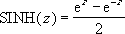## Excel Office

Excel How Tos, Tutorials, Tips & Tricks, Shortcuts

# SINH function: Description, Usage, Syntax, Examples and Explanation

## What is SINH function in Excel?

SINH function is one of the Math and Trig functions in Microsoft Excel that returns the hyperbolic sine of a number.

## Syntax of SINH function

SINH(number)

The SINH function syntax has the following arguments:

• Number: Any real number.

## SINH formula explanation

The formula for the hyperbolic sine is:## Example of SINH function

Steps to follow:

1. Open a new Excel worksheet.

2. Copy data in the following table below and paste it in cell A1

Note: For formulas to show results, select them, press F2 key on your keyboard and then press Enter.

You can adjust the column widths to see all the data, if need be.

 Formula Description Result =2.868*SINH(0.0342*1.03) Probability of obtaining a result of less than 1.03 seconds. 0.1010491